# View item statistics

Item statistics in the Gradebook are data on how your students performed on all pieces of content within a specific activity.

After performing a Gradebook search (check out Search for class grades), you can view this statistical data while viewing activity Gradebook data (check out View activity Gradebook data).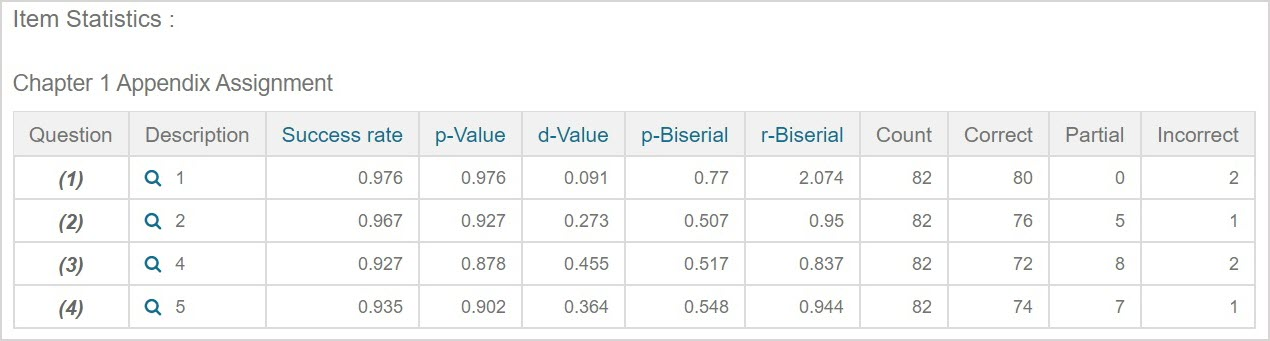NOTE: In order for item statistics to be calculated, the content must be accessible to a student by being contained within a visible activity.

TIP: You can view item statistics for individual pieces of content across all activities that the content is used in through the Content Summary pane of the Content Repository. Check out View the Content Summary pane.

IMPORTANT: Item statistics—when viewed using the Gradebook—can differ from the statistics calculated in the Content Summary pane within the Content Repository. This is because the Gradebook captures all attempts at the piece of content within a specific activity, whereas the Content Repository captures all attempts at the piece of content across all activities that it's contained in (the latter may not differentiate between student levels of mastery). Example — If a question about a newly-learned concept is first attempted on an introductory assignment, the students viewing this question may find it more difficult and perform less optimally than when it is presented on a subsequent review assignment. Therefore, the statistics displayed in the Content Repository's Content Summary pane will be less specific to the student level of mastery completing the activity than when they are viewed using the Gradebook's Item Statistics page.

## View item statistics

To view the item statistics for all of the content in a specific activity:

1. Click Gradebook on the Class Homepage.1. Click Item Statistics.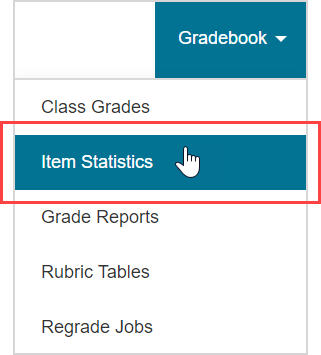1. Select the unit where the activity of interest is stored from the Units list.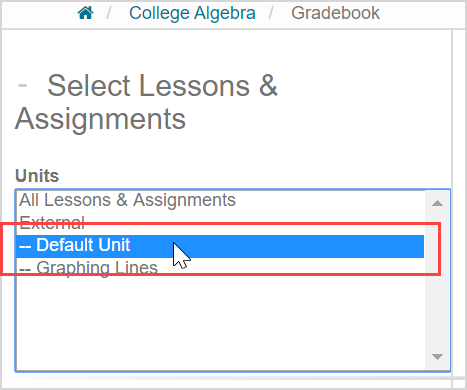TIP: Select All Lessons & Assignments to display all of your class's activities in the list of available activities.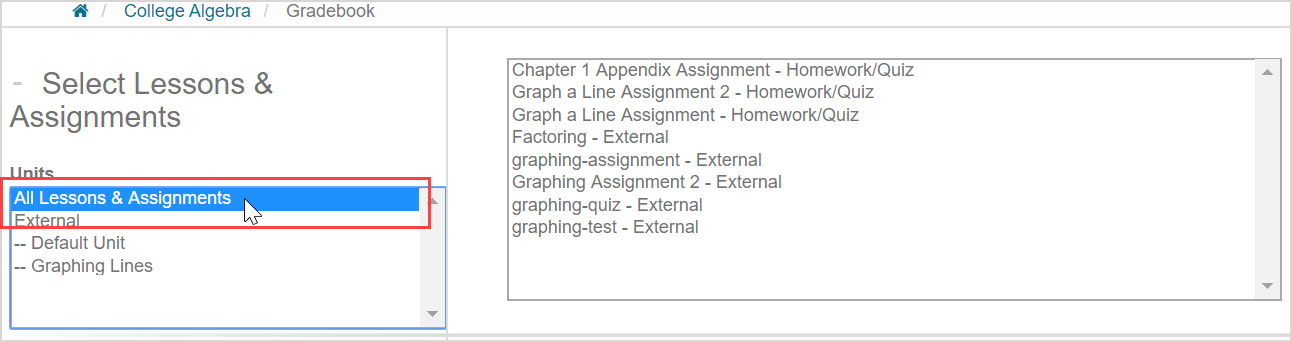TIP: You can select more than one unit by pressing Ctrl (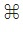) and clicking on the units you want to include in your search.

1. Select the specific activity of interest from the list of available activities.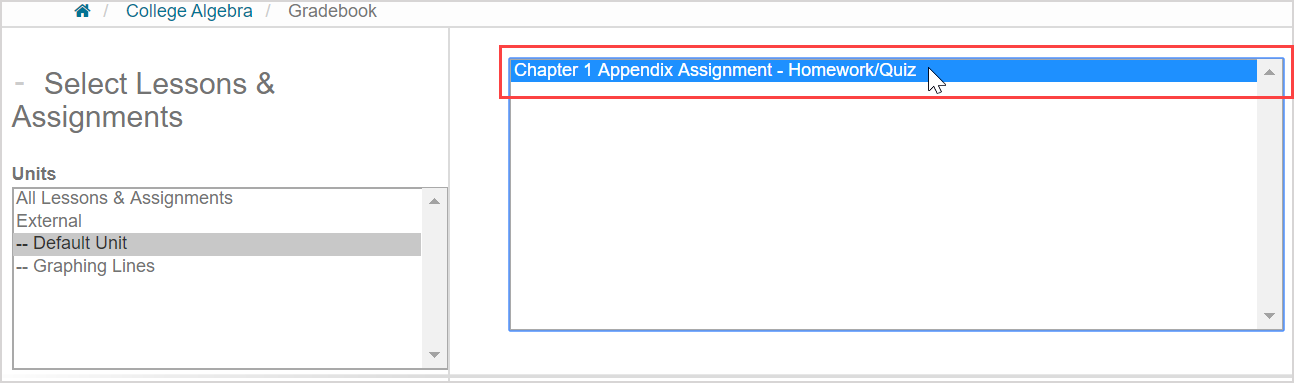TIP: You can select more than one activity by pressing Ctrl () and clicking on the activities you want to include in your search.

1. Select which class you want to use to view the item statistics from the Classes list in the Filter Results pane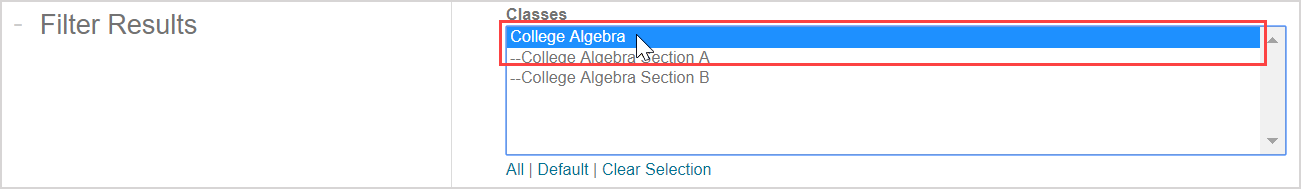TIP: The parent class and all associated child classes will appear in the Classes list. You can select more than one class for viewing item statistics. Press Ctrl () and continue to select other classes to select more than one class or child class.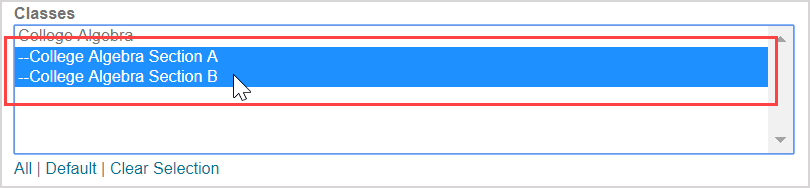Alternatively, you can:

• Click All to select all of the classes in the Classes list.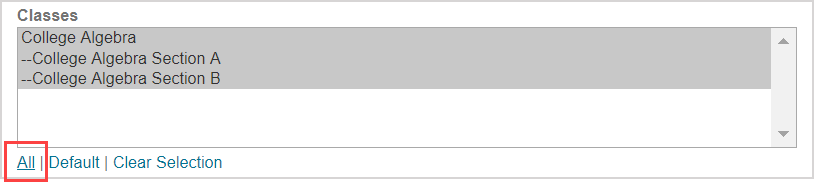• Click Default to only select the parent class in the Classes list.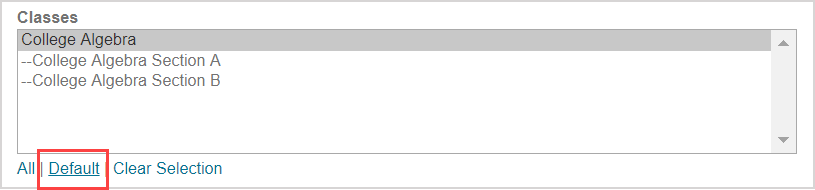• Click Clear Selection to deselect all classes in the Classes list.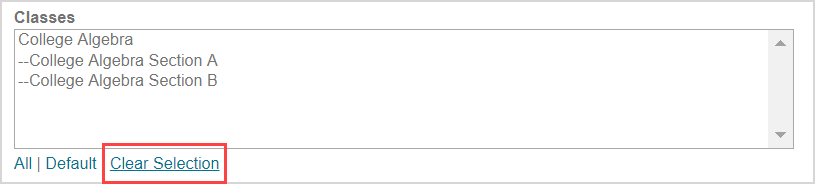1. (Optional) Customize the data fields beyond the default settings that control what information is included in the calculations of your item statistics. Check out Use additional Gradebook search criteria.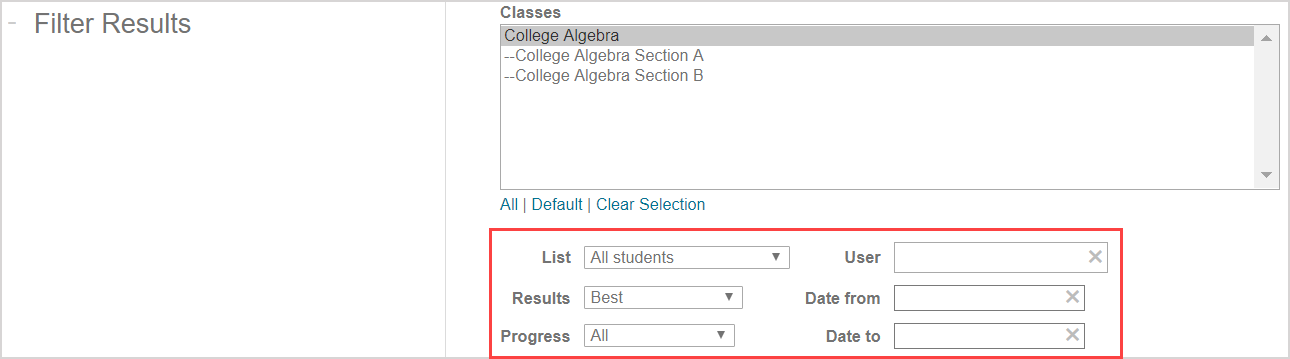1. Click Search.1. The item statistics are displayed as a part of the activity's/activities' Gradebook data (check out View activity Gradebook data for more details on other information that's displayed on this page).TIP: Click on the preview icon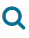to be reminded of the question content.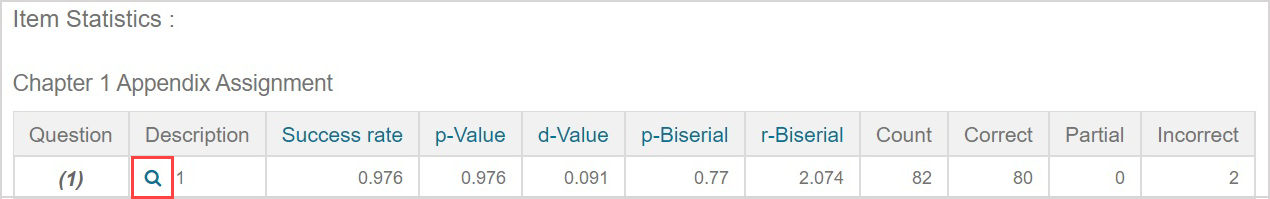Click Close in the question preview to return to the item statistics.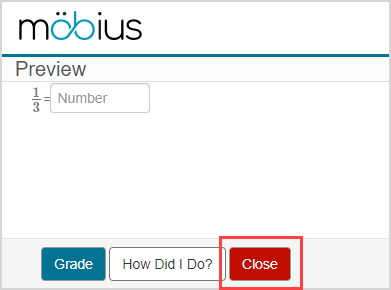NOTE: If you edited the activity after students have started their attempts, this can affect the accuracy of the item statistics results and you should interpret the results with caution. This interference is indicated with the caution icon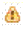.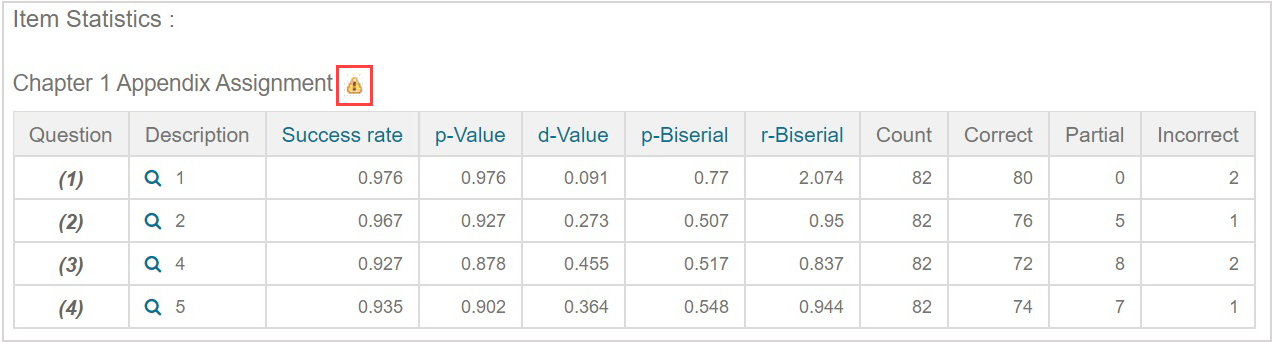NOTE: If your activity includes a question group, questions within the question group are displayed under a single question number (Example(4)). Each question within the question group is then numbered (Example1, 2, and 3 within the question group identified as question (4)).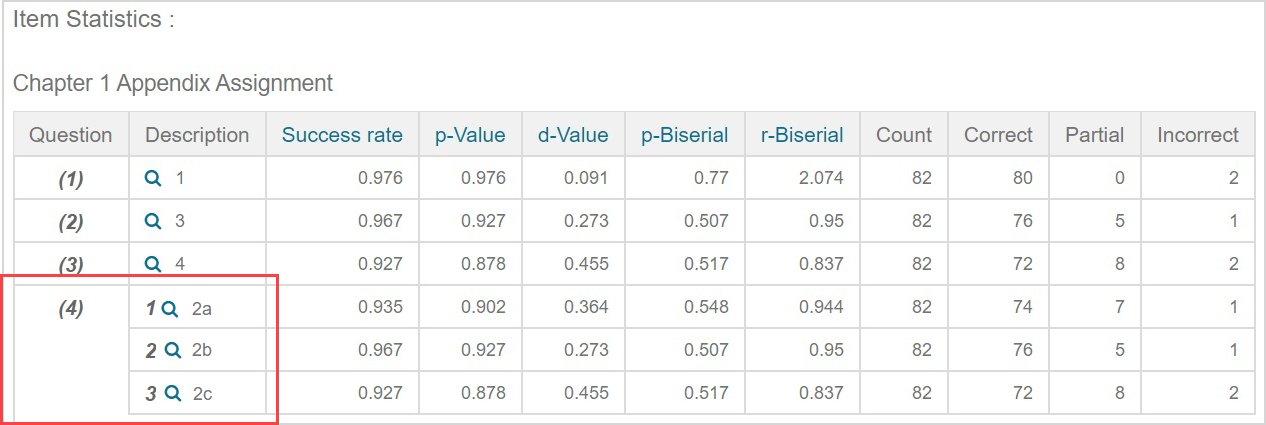## Item statistic calculations

The numerical values of the item statistics are calculated using these formulas:

TIP: Click each statistic heading in the results table for a pop-up that explains how the value was calculated.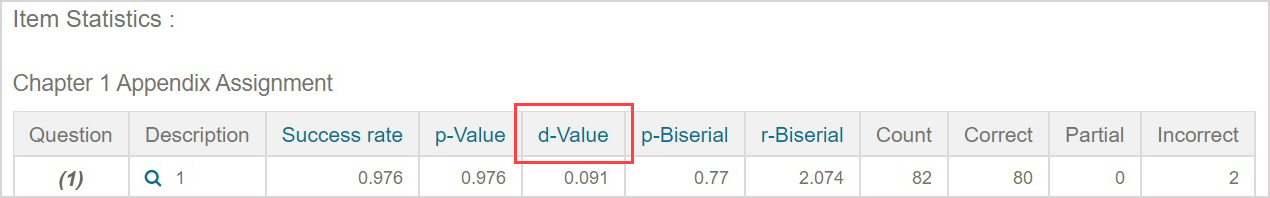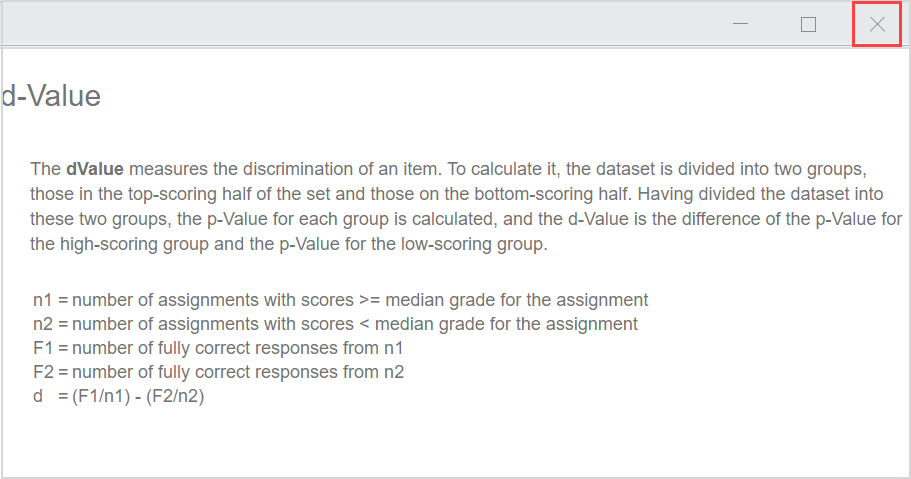Statistic

Explanation

Success Rate

The average normalized score on the item.

With each item graded between 0.0 and 1.0 (normalized), the average score is computed over the set of all scores recorded for that item.

p-Value

The ratio of the number of fully correct responses to the total number of responses in the data set.

A question is deemed to be fully correct only if it has a score of 1.0/1.0.

• p = F/n
• F = number of fully correct responses
• n = number of responses
d-Value

The discrimination of an item.

The dataset of scores is divided into two groups: those in the top-scoring half of the set and those on the bottom-scoring half.

The p-Value for each group is calculated.

The d-Value is the difference of the p-Value between the two groups.

• d = (F1/n1) - (F2/n2)
• F1 = number of fully correct responses from n1
• F2 = number of fully correct responses from n2
• n1 = number of activities with scores >= median grade for the activity
• n2 = number of activities with scores < median grade for the activity
p-Biserial

A correlation coefficient relating the performance on an item and performance on the total activity.

An index of discrimination that measures the extent to which students who score high on the activity tend to get the item correct and those who score low tend to get the item incorrect.

•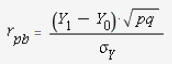• Y1 = mean grade of activities on which this item was answered correctly (1.0).
• Y0 = mean grade of activities on which this item was answered incorrectly.
• p = p-Value
• q = 1-p
•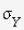= standard deviation for activity grades.
r-Biserial

A correlation coefficient relating performance on an item and performance on the total activity.

An index of discrimination that measures the extent to which students who score high on the activity tend to get the item correct and those who score low tend to get the item incorrect.

•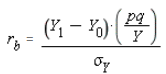• Y1 = mean grade of activities on which this item was answered correctly (1.0).
• Y0 = mean grade of activities on which this item was answered incorrectly.
• p = p-Value
• q = 1-p
• Y = the height of the standardized normal distribution at the point z, where P(z'<z)=q and P(z'>z)=p.
•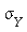= standard deviation for activity grades.
CountThe number of times students have attempted this question.
CorrectThe number of times a student has answered this question and received a score of 1.0/1.0.
PartialThe number of times a student has received a partial mark (0.0 < x < 1.0/1.0) for this question.
IncorrectThe number of times a student has received a score of 0.0/1.0 for this question.